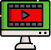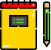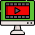VideosNotes

### Approximation

LOGARITHMS
Logarithm, the exponent or power to which a base must be raised to yield a given number.
Views: 1
Video Duration: 4:12
On the Same TopicLogarithmic Equations
i. Revision of logarithm of...Anti-Logarithm
The anti-logarithm of a num...Logarithm - Quotient Rule
Since eln(x/y)=eln(x)−ln(y)...LOGARITHMS NUMBERS
Logarithm, the exponent or ...
Other Related Videos